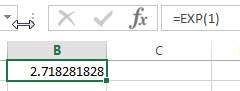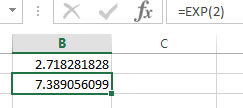# Excel EXP Function

This post will guide you how to use Excel EXP function with syntax and examples in Microsoft excel.

### Description

The Excel EXP function returns e raised to the power of a number.  The constant e is equal to 2.71828182845904, and it is the base of the natural logarithm. So you can use EXP function to get the value of e raised to the power of a given number.

The EXP function is a build-in function in Microsoft Excel and it is categorized as a Math and Trigonometry Function.

The EXP function is available in Excel 2016, Excel 2013, Excel 2010, Excel 2007, Excel 2003, Excel XP, Excel 2000, Excel 2011 for Mac.

### Syntax

The syntax of the EXP function is as below:

`= EXP (number)`

Where the EXP function argument is:

• number –This is a required argument. The power to raise e to.

Note:

• To calculate powers of other bases, use the exponentiation operator (^).
• EXP is the inverse of LN, the natural logarithm of number.

### Excel EXP Function Examples

The below examples will show you how to use Excel EXP Function to return value of the constant e raised to a given number.

1# get the approximate value of e, enter the following formula in Cell B1.

`=EXP(1)`2# get the base of the natural logarithm e raised to the power 2, enter the following formula in Cell B2.

`=EXP(2)`